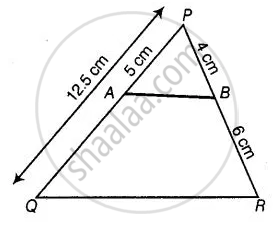# A and B are respectively the points on the sides PQ and PR of a triangle PQR such that PQ = 12.5 cm, PA = 5 cm, BR = 6 cm and PB = 4 cm. Is AB || QR? Give reasons for your answer. - Mathematics

Sum

A and B are respectively the points on the sides PQ and PR of a triangle PQR such that PQ = 12.5 cm, PA = 5 cm, BR = 6 cm and PB = 4 cm. Is AB || QR? Give reasons for your answer.

#### Solution

Given, PQ = 12.5 cm, PA = 5 cm, BR = 6 cm and PB = 4 cmThen QA = QP - PA = 12.5 - 5 = 7.5 cm

Now, (PA)/(AQ) = 5/7.5 = 50/75 = 2/3  ......(i)

And (PB)/(BR) = 4/6 = 2/3  ......(ii)

From equations (i) and (ii), (PA)/(AQ) = (PB)/(BR)

By converse of basic proportionally theorem,

AB || QR

Concept: Basic Proportionality Theorem (Thales Theorem)
Is there an error in this question or solution?

#### APPEARS IN

NCERT Mathematics Exemplar Class 10
Chapter 6 Triangles
Exercise 6.2 | Q 3 | Page 63

Share